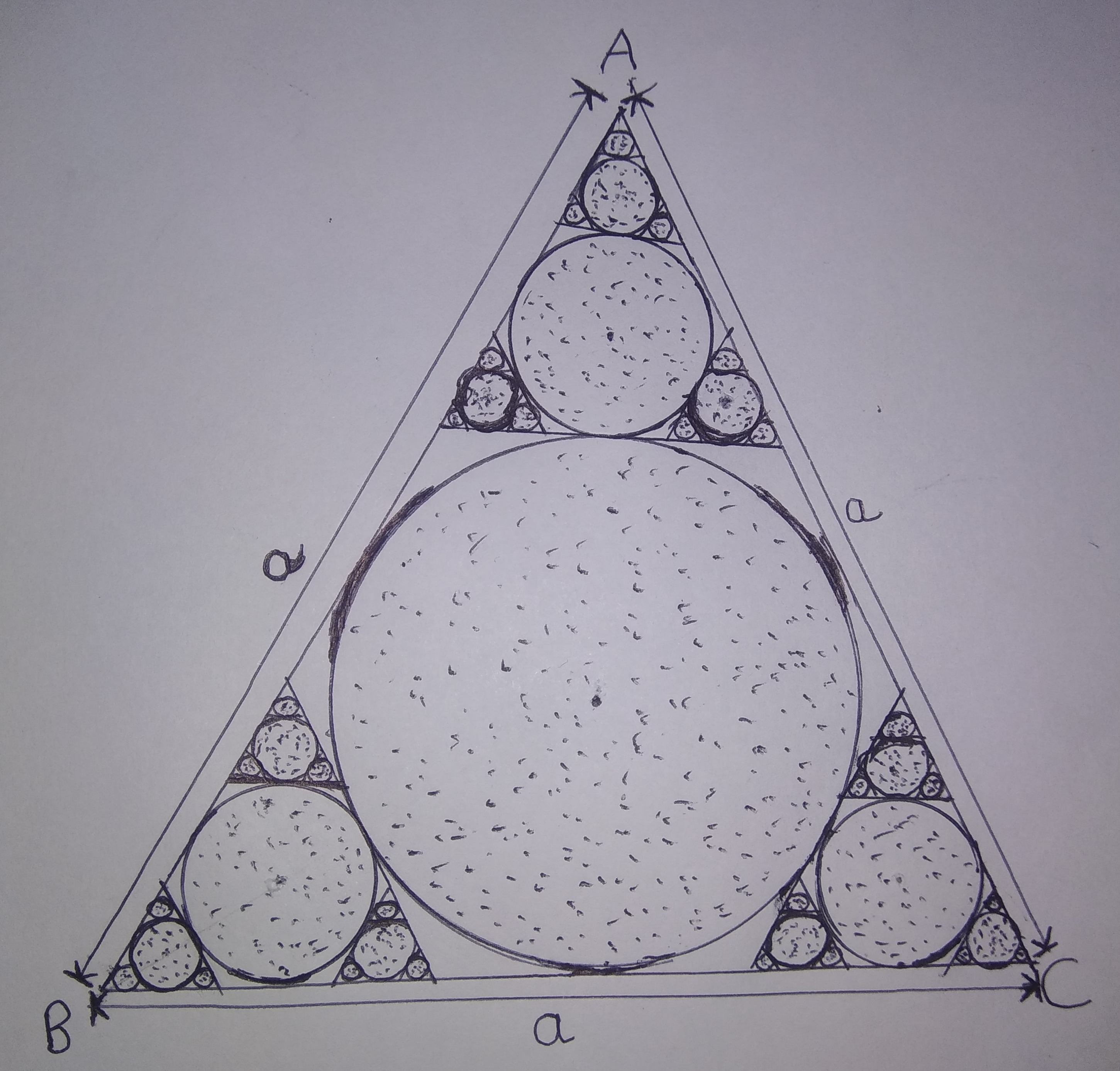# Infinite Geometrical Geometric Series!

Geometry Level 4Above figure consists of $\color{#3D99F6}{\infty \text{ circles}}$ inscribed in an $\color{#3D99F6}{\text{Equilateral Triangle}}$ in a pattern as shown above. Let $\color{#3D99F6}{\text{Area of Infinite circles} = A_1}$$(\text{dotted region})$ and $\color{#3D99F6}{\text{Area of Equilateral Triangle } \Delta ABC= A_2}$.

Find value of $\color{#3D99F6}{\frac{A_1}{A_2}}$. If your answer is of form $\color{#3D99F6}{\frac{\pi}{\sqrt{x}}}$, enter answer as $\color{#3D99F6}{x}$.

All of my problems are original

×